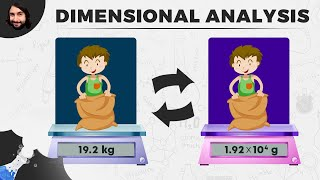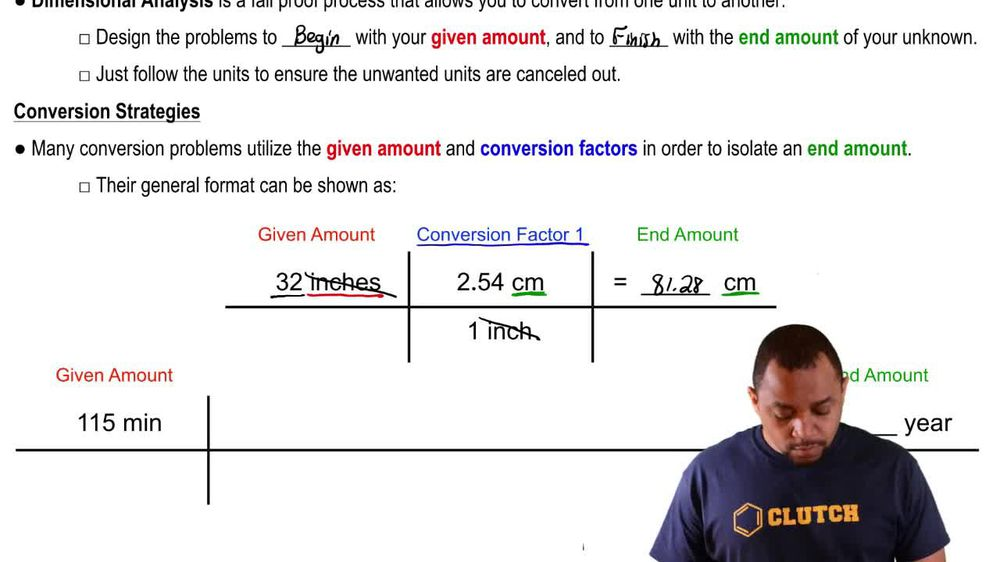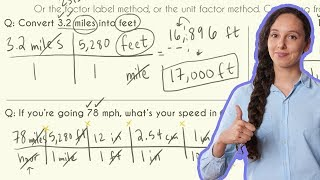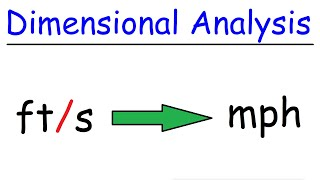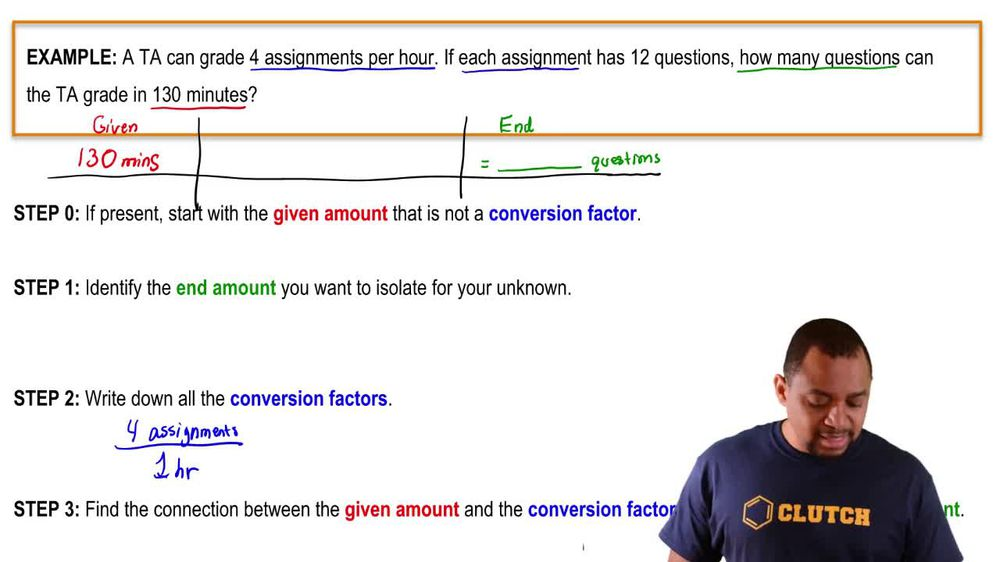Start typing, then use the up and down arrows to select an option from the list.
1. 1. Intro to General Chemistry2. Dimensional Analysis
Problem

# Ocean currents are measured in Sverdrups (sv) where 1 sv = 109 m3/s. The Gulf Stream off the tip of Florida, for instance, has a flow of 35 sv. (c) How much time is required for 1 petaliter (PL; 1 PL = 1015 L) of seawater to flow past a given point?

Relevant Solution1m
Play a video:
Hey everyone, we're told that a hypothetical measurement terms 50 is used to measure water currents where once, fifth is equal to 14 m3 per minute. If a freshwater river has a flow of nine point 8/5 how long does it take a mega liter of fresh water to pass a specific point? And they provided us our conversion factor from mega leader into leaders. To answer this question, we first need to convert our 9.850 into m3 permanent. Using our dimensional analysis, we know that one's 5th Contains 14 m3/min. So when we calculate this out and cancel out our unit, we end up with 137.2 cubic meters per minute, which we will use to answer our question. Now, since our question asks us, how long does it take a mega liter of fresh water to pass a specific point. This means we'll have to start off with one mega liter. Now we know that we have one mega liter and this contains 10 to the six leaders and we also have to remember another conversion factor which is 10 to the third leaders is equivalent to one cubic meter. And as we calculated previously, we have 137.2 m3 per one minute. So when we calculate this out, We end up with a value of 7.289 minutes, Which we can round 2 7.3 minutes and this is going to be our final answer. Now I hope that made sense and let us know if you have any questions